# Multi-digit Subtraction Math Drill Worksheets

The worksheets in this section range from simple subtraction of 2-digit numbers to subtracting large numbers with multiple regroupings.

Let’s take you through the worksheets available.

## Subtraction without regrouping

There are four sets of multi-digit subtraction with no regrouping worksheets in this section:

• 2 to 3-digit minus 1-digit worksheets with no regrouping
• 2-digit minus 2-digit worksheets with no regrouping
• 3-digit minus 3-digit worksheets with no regrouping
• 4-digit minus 4-digit worksheets with no regrouping

This is what the 2-digit minus 2-digit worksheet with no regrouping looks like: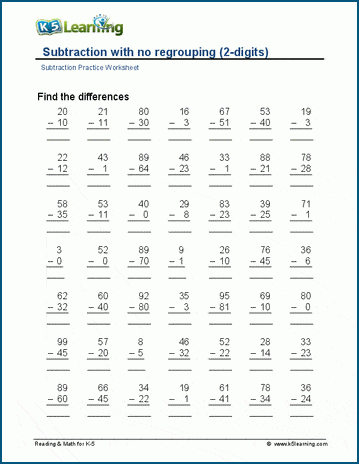## Multi-digit subtraction with regrouping

Following is a set of worksheets that focus on drilling regrouping in multi-digit subtraction:

• 2-digit minus 1-digit subtraction with regrouping
• 2-digit minus 2-digit subtraction with regrouping
• 3-digit minus 2-digit subtraction with regrouping 3-digit minus 3-digit subtraction with regrouping
• Subtracting up to 4 digits with regrouping
• Subtracting up to 5 digits with regrouping
• Subtracting up to 8 digits with regrouping

This worksheet covers subtracting up to 8 digits with regrouping.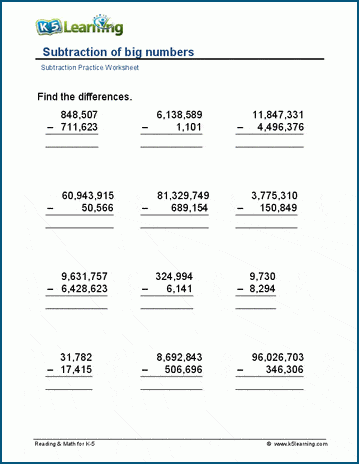## Horizontal subtraction practice

Next, we tackle multi-digit subtraction on the horizontal. This is a mental math exercise:

• Horizontal subtraction with no regrouping
• Horizontal subtraction with regrouping
• Horizontal subtraction of up to 2-digit numbers
• Horizontal subtraction of up to 3-digit numbers

The 2-digit horizontal subtraction worksheet looks like this: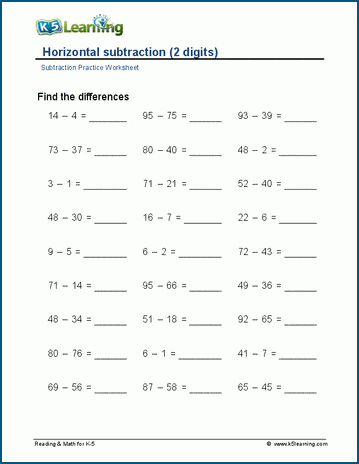## Subtraction tables

These subtraction tables help students drill multi-digit subtraction in a concise way. We have two sets of worksheets: 1-digit subtrahends and 2-digit subtrahends.

This table shows the 2-digit subtrahend exercise: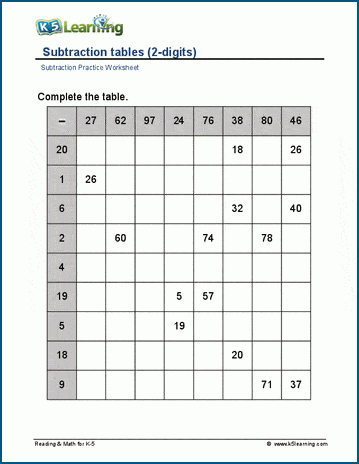## Worksheets covering missing minuends and subtrahends

The next level of difficulty is working through exercises of missing minuends and subtrahends. These worksheets are organized as follows:

• Subtraction with missing numbers (no regrouping)
• Subtraction with missing numbers (with regrouping)
• Subtraction with missing numbers (2-digits)
• Subtraction with missing numbers (3-digits)

This worksheet has students working on missing numbers with regrouping: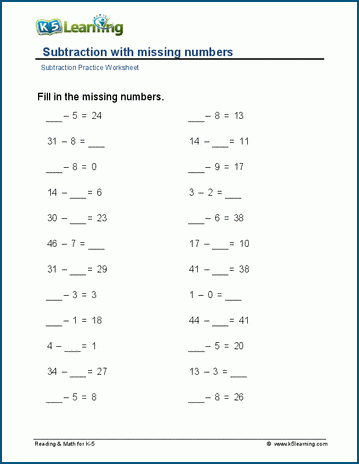## Subtracting tens, hundreds and thousands practice

The final section of worksheets covers the subtraction of tens, hundreds and thousands:

• Subtracting from 10, 100 and 1,000
• Subtracting from multiples of 10
• Subtracting from multiples of 100
• Subtracting from multiples of 1,000
• Subtracting from zeros.

In this worksheet, students are working subtracting from multiples of 100.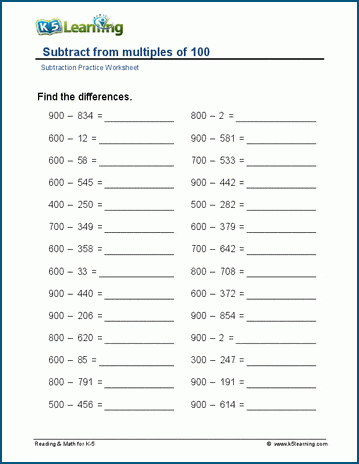Become a Member

This content is available to members only.